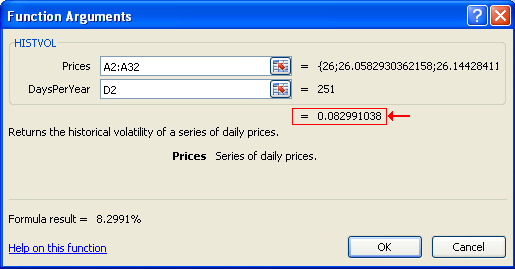XLL+ Class Library (7.0)

# Avoiding the Excel Formula Wizard

From the developer's point of view, the most dangerous aspect of the Formula Wizard is the preview result that appears in the palette, as shown below.If you have a function that takes a long time to calculate, you may want to avoid calculating it for the preview in the Formula Wizard. It will be called every time the user changes an argument field, which is a waste of time until the user has finished filling in all the arguments.

You can use the CXllApp::IsInFormulaWizard() method to find out if the formula is being called from the Formula Wizard.

The example shown below returns #N/A! if the it is being called from the Formula Wizard. This shows up as a blank in the Excel Formula Wizard.CopyC++
```CXlOper* SLOWCALC_Impl(CXlOper& xloResult, double X, double Y)
{
// End of generated code
//}}XLP_SRC
if (CXllApp::IsInFormulaWizard())
xloResult = xlerrNA;
else
xloResult = LongSlowFunction(X, Y);
return xloResult.Ret();
}```